# How to Stop Formulas Auto Calculation in Excel

Normally we often use auto calculation in excel to do sum or other formulas for convenient. But sometimes we want to stop this after several calculations. In below article you will have two ways to stop the auto calculation. The first way is stop auto calculation by formulas options in Excel directly. The other way is by VBA.

First see an auto calculation example below:

Set two lists of numbers in A and B columns, C column is the total of A+B. Enter “=A1+B1” in C1, then the result is auto calculated.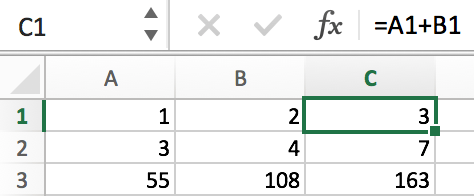Select C1 and drag it down to C2 and C3, then C2 and C3 are auto calculated correctly.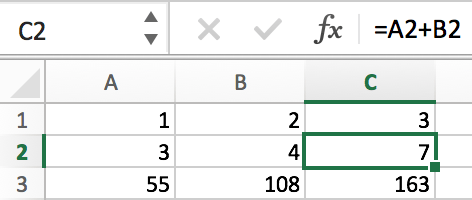Let’s start to learn how to stop auto calculation now.

## Method1: Stop Auto Calculation by Excel Calculation Options

Step 1: In condition, we add a new combination of numbers in A4 and B4.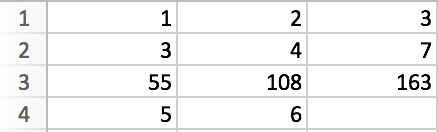If you don’t do anything, and just drag down C3 to C4, you will get 11 directly. This is because the auto calculation is not stopped. So we need to stop the calculation now.

Step2: Select C3, click on Formulas menu. Then Formulas related options are loaded.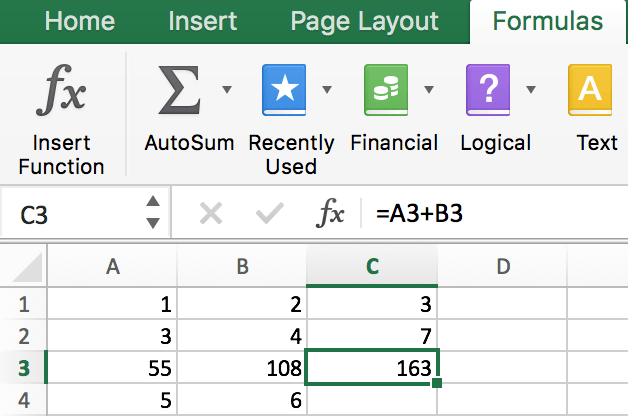Step 3: Click on Calculation Options in the right of the bar.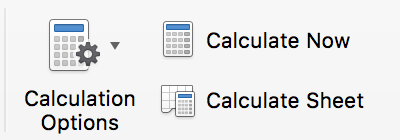Step 4: Click on the dropdown list, select Manual.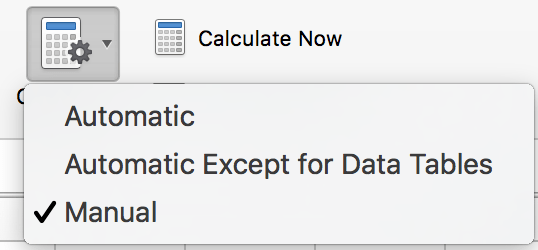Step 5: Then drag down C3 to C4, you will get 163 which is just copy the number from C3 and will not execute the calculation.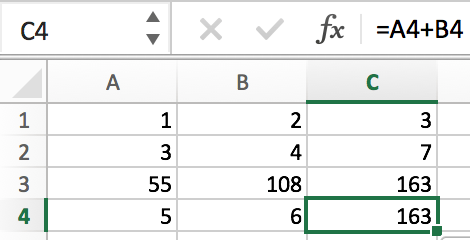See, this way is very useful for doing manual re-calculate if customer required. Now let’s get started to learn another way to stop auto calculation.

## Method2: Stop Auto Calculation by VBA

You can also use an Excel VBA macro to achive the same reusult. and  just do the following steps:

Step1: right click on your current worksheet tab in the bottom of your worksheet, and right click on it, and then select view code menu from the context menu list.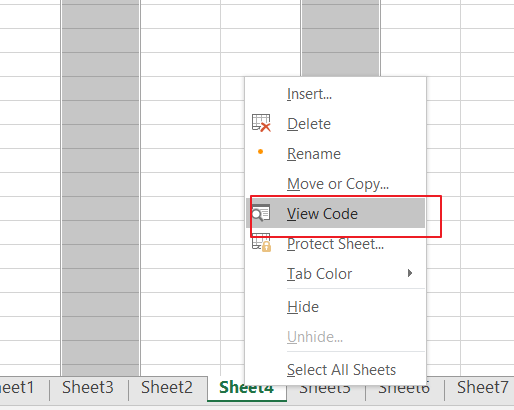Step2: the Visual Basic for Application window will appear. and click Insert tab, and select module from the drop down menu list.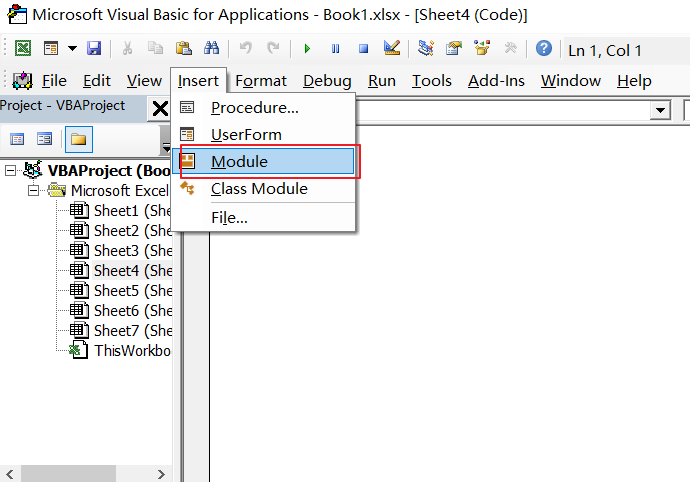Step3: copy the below vba code into code window.  save and close VBA window.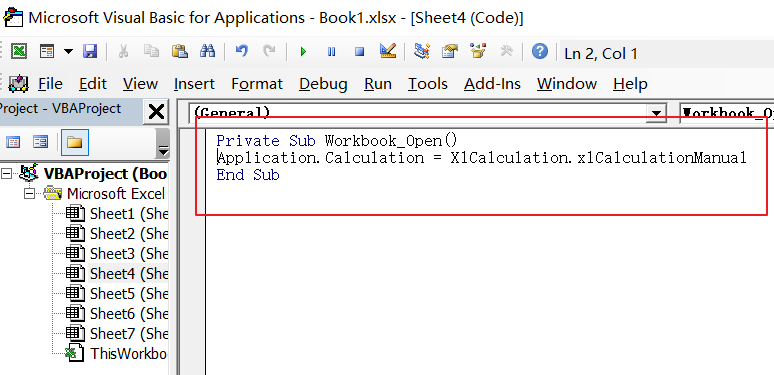Step4: you need to reopen your workbook to make it affect.

Related Posts

VBA Macro For VLOOKUP From Another Sheet

In the previous post, you should know that how to fix or remove the #N/A error when using VLOOKUP formula to lookup value from another sheet. And this post will show you how to use VBA code to vlookup data ...

How To Insert Comments in Protected Worksheet in Excel

This post will show you how to allow comments in a protected worksheet in Excel. You can easily to insert comments into cells in a normal worksheet in Excel, but if want to insert a comment in a worksheet that ...

How To Convert Text to Upper Cases(Using VBA) in Excel

This post will show you how to switch from lower case to upper case in Excel. and I am going to show you two different ways of converting text to upper cases using formula or VBA macro in Excel 2013,Excel ...

How To Hide Every Other Row in Excel (Using VBA)

This post will show you how to hide alternate rows or columns in Excel or how to hide every third, fourth, fifth row or column in Excel. If you want to hide every other row in your current worksheet, how ...

How to Disable the Save As Prompt in Excel

This post will show you how to use a VBA Macro to save an Excel file and overwrite any existing file without a prompt so that you are going to get the little window that says file already exists do ...

How to Count Cells that Contain even or odd numbers in Excel

This post will guide you how to count the number of cells that contain odd or even numbers within a range of cells using a formula in Excel 2013/2016.How do I count cells that contain odd numbers through the use ...

How to Count Cells that Contain negative Numbers in Excel

This post will guide you how to count the number of cells that contain negative numbers within a range of cells using a formula in Excel 2013/2016.You can count the number of negative numbers in your data using easy functions ...

How to Count Cells Are Not Blank or Empty in Excel

This post will guide you how to count cells that are not blank or empty in a given range cells using a formula in Excel 2013/2016.How do I count the number of cells that are not blank in a particular ...

How to Count Cells Not equal to X or Y in Excel

This post will guide you how to count the number of cells not equal to criteria X or Y in a given range cells using a formula in Excel 2013/2016.You can easily to count cells equal to or not equal ...

How to Count Cells Less Than a Specific Value in Excel

This post will guide you how to count the number of cells less than a particular numeric value in a given range cells using a formula in Excel 2013/2016. How do I count cells that are less than a specific ...

Sidebar